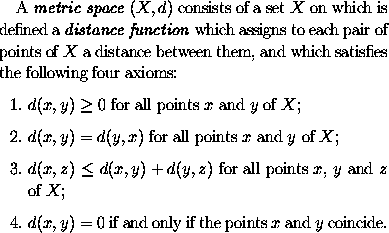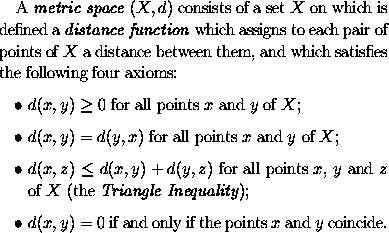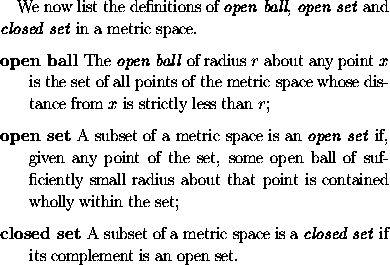### Lists

LaTeX provides the following list environments:

• enumerate for numbered lists,
• itemize for un-numbered lists,
• description for description lists

Numbered lists are produced using

\begin{enumerate} ... \end{enumerate}

The items included in the list should be sandwiched between \begin{enumerate} and \end{enumerate} and should each be preceded by the control sequence \item (which will automatically generate the number labelling the item). For example, the textis generated by LaTeX from the following input:
A \emph{metric space} $(X,d)$ consists of a set~$X$ on
which is defined a \emph{distance function} which assigns
to each pair of points of $X$ a distance between them,
and which satisfies the following four axioms:
\begin{enumerate}
\item
$d(x,y) \geq 0$ for all points $x$ and $y$ of $X$;
\item
$d(x,y) = d(y,x)$ for all points $x$ and $y$ of $X$;
\item
$d(x,z) \leq d(x,y) + d(y,z)$ for all points $x$, $y$
and $z$ of $X$;
\item
$d(x,y) = 0$ if and only if the points $x$ and $y$
coincide.
\end{enumerate}


Un-numbered lists are produced using

\begin{itemize} ... \end{itemize}

Thus if we replace \begin{enumerate} and \end{enumerate} in the above input by \begin{itemize} and \end{itemize} respectively, LaTeX generates an itemized list in which each item is preceeded by a bullet':Description lists (for glossaries etc.) are produced using
\begin{description} ... \end{description}

The items included in the list should be sandwiched between \begin{description} and \end{description} and should each be preceded by \item[label], where label is the label to be assigned to each item. For example, the textis generated by LaTeX from the following input:
We now list the definitions of \emph{open ball},
\emph{open set} and \emph{closed set} in a metric space.
\begin{description}
\item[open ball]
The \emph{open ball} of radius~$r$ about any point~$x$
is the set of all points of the metric space whose
distance from $x$ is strictly less than $r$;
\item[open set]
A subset of a metric space is an \emph{open set} if,
given any point of the set, some open ball of
sufficiently small radius about that point is contained
wholly within the set;
\item[closed set]
A subset of a metric space is a \emph{closed set} if its
complement is an open set.
\end{description}
`